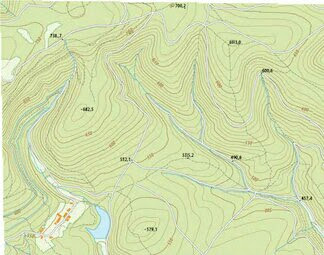WHAT IS THE USE OF SURVEYING AND LEVELING IN THE ENGINEERING

# WHAT IS THE USE OF SURVEYING AND LEVELING IN THE ENGINEERING

UPENDRA KUMAR

WHAT IS THE SURVEYING AND LEVELING

SURVEYING:- Surveying is the art for determining the relative position of different objects on the earth's surface by measuring a horizontal distance between them. The aim of surveying is to prepare a map on a suitable scale.

LEVELING:-Leveling is the art of determining the relative vertical distance of different points.

CLASSIFICATION OF SURVEYING

PLANE SURVEYING
GEODETIC SURVEYING

PLANE SURVEYING

In the plane surveying, the curvature of the earth is not taken into consideration. The plane surveying used in a small area, so the surface of the earth considered as the plane. The line joining between two points considered straight. The triangle formed by any three points considered as a plane triangle. The angle measured between the lines of the triangle assumed the plane angle. The plane surveying is used were to survey area less than 2.5 km square.

GEODETIC SURVEYING

In the geodetic surveying, the curvature of the earth is taken into consideration. The geodetic surveying used in a large area, so the surface of the earth considered spherical. The line joining between two points considered curved. The triangle formed by any three points considered a spherical triangle. The angle measured between the lines of the triangle assumed the spherical angle. The geodetic surveying is used were to survey area less than 2.5 km square.

There are two methods of surveying:
A. Triangulation surveying. B. Traverse surveying.

Types of surveying based on the instrument.
i.Chain Surveying
ii.Compass Surveying
iii.Plane Table Surveying
iv.Theodolite Surveying
iv.Tacheometric Surveying
v.Photographic surveying.
The Theodolite and Tacheometric surveying are also used in leveling purposes.
Types of surveying based on modern instruments.

i. DGPS Surveying
ii. Total Station Surveying
iii. 3D Scanning Surveying
iv. Drone Surveying3D scanner

The modern instruments are used in surveying and leveling purposes.

Uses Of Surveying And Levelling In Engineering

The surveying and leveling are used to prepare a detailed drawing of projects involving roads, railway, etc.
Types of Branch of Engineering where The Use of Surveying
1. Civil Engineering
2. Mining Engineering
3.Marine Engineering
4.Astronomical Engineering.Topographic Map

The following method of survey used in engineering.
a. Topographic surveying:- To determine the natural features of the country.
b. Cadastral Surveying:- To determine the boundary and detail of the field, estate, and houses.
c.Construction and layout Surveying:- It is used in construction, quantity, and layout work of engineering projects.
d.  Archeological surveying